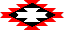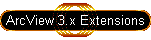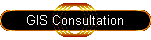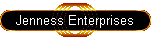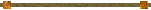#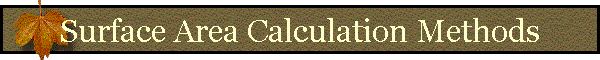In summary, this extension calculates surface areas using the following strategy: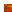For each cell in the grid, surface areas are based on triangle areas derived from eight triangles.Each triangle connects the center point of the central cell with the center points of two adjacent cells.  These triangles are located in three-dimensional space, so that the area of the triangle represents the true surface area of the space bounded by the three points.The triangle area is adjusted so that it only represents the portion of the triangle that overlays the central cell.The areas of the eight triangles are summed to produce the total surface area of that cell.The surface ratio of the cell is calculated by dividing the surface area of the cell with the planimetric area of the cell.

For More Detail:  This extension derives surface areas for a cell by using information from the eight cells adjacent to the center cell.  For example, given a sample elevation grid: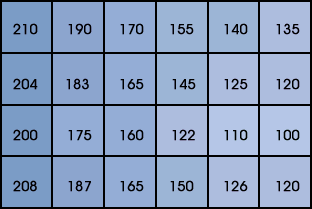this extension would calculate the surface area for the cell with elevation value "183" based on the elevation values of the eight cells surrounding it,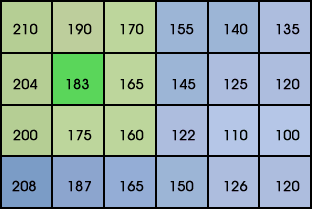and the surface area for the "165" cell based on the eight elevation value surrounding it: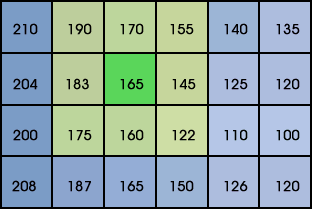The following example of a Surface Area calculation will be based on the illustration above, where we will calculate the surface area for a cell with an elevation value of 165, surrounded by cells at 190, 170, 155, 183, 145, 175, 160 and 122.  This grid of elevation values can be pictured In 3-dimensional space as a set of adjacent columns, each rising as high as it's specified elevation value.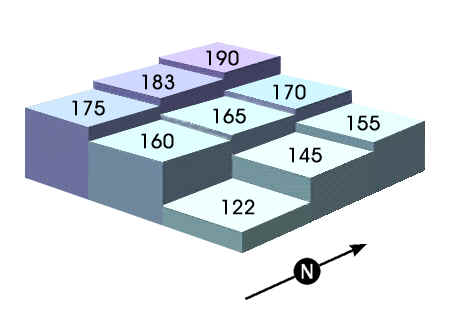We take the 3-dimensional centerpoints of each of these 9 cells, and calculate the lengths of the 8 lines that connect the central cell's centerpoint with the center points of the 8 surrounding cells.  We then calculate the lengths of the lines that connect each of the 8 surrounding cells with the one right next to it, so that we end up with the lengths of the sides of the 8 triangles that all meet at the center point of the central cell.  Using these lengths, we can calculate the areas of each of the triangles.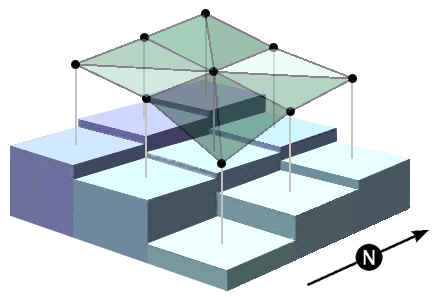These lengths are simple to calculate using the Pythagorean Theorem, which states that, in a right triangle, the square of the length of the hypotenuse is equal to the sum of the squares of the other two sides.  Thus, for any two points A and B: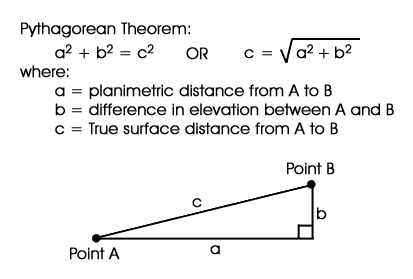"b" is easy to calculate, since it is simply the difference between the two cell values.  "a" is easy to get for the cells directly to the North, East, South and West, since it is simply the value of the Cell size.  For the diagonals, we use the Pythagorean theorem again and calculate that "a" is equal to the square root of (2) x (a-squared).

However, since we're only concerned with the surface area within each cell, we cut all the triangle lengths in half.  Now when we calculate the area of a triangle based on the lengths of three sides, the three sides will represent the portion of the triangle that lies within the cell boundaries.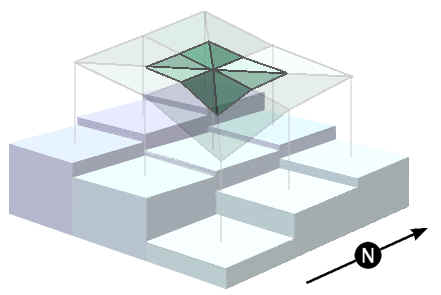Technical Note:  The above description only describes the logic used to derive the surface area.  The extension is not actually connecting any lines or using any vector data at all.  Rather, the extension creates 16 new grids, where the cell values represent the lengths of one of the 16 triangle edges pictured above.  Using 3 of these 16 "Triangle Edge Length" grids at a time, it then calculates 8 new grids representing the area of the triangle that would be bounded those three edge lengths.  Finally, the extension adds up those 8 "Triangle Area" grids to produce the final Surface Area Grid.Calculating the Area of a Triangle from the Lengths of the Three Sides:  The author is aware of two formulae that will produce the area of a triangle given the lengths of the three sides, and will describe both of them here.  The first one was derived by the author from a couple of basic mathematical theorems and the second was discovered by the author after he figured out the first one.  This extension uses the first one because it was all worked out by the time the author found out about the second formula.  The author has checked to confirm that these two formulae produce identical results.

Formula 1:  Derived by the author -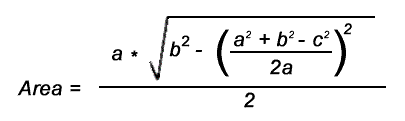Formula 2:  Adapted from the "Triangle Area Calculator" (click to visit web site), and attributed to Heron of Alexandria and Archimedes (see also Abramowitz and Stegun [1972, p. 79]):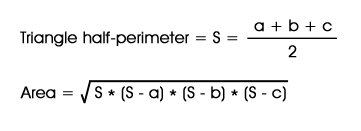Derivation of Formula 1:

1)  Given a triangle with sides a, b, and c: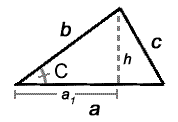and the standard formula for the area of a triangle: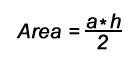The main problem was to figure out what h was equal to, given that we knew what a, b, and c were.

2)  The Law of Cosines is useful for identifying the length of a third side if you know the lengths of two sides and the angle between them: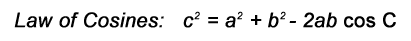3)  Since we know the three sides, we can isolate "cos C" on one side of the equation: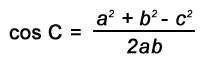4)  In a right triangle (see triangle illustrated above, but look at sides a1, h, and b), cos C is also equal to the adjacent side divided by the hypotenuse: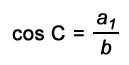5)  Therefore,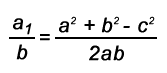6)  Multiplying both sides by b, we now have isolated a1: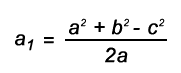7)  We can use the Pythagorean Theorem to isolate h:

 Pythagorean Theorem: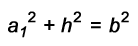- or -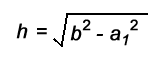8)  Substituting for a1: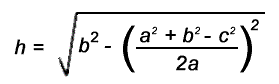9)  Finally, plugging h back into the Area equation, we get:REFERENCES:

Abramowitz, M., and I. A. Stegun. 1972. Handbook of mathematical functions with formulas, graphs and mathematical tables. Dover Publications, Inc. New York, New York, USA.Jeff Jenness

3020 N. Schevene Blvd.

Flagstaff, AZ  86004

USAMore Online Documentation for " Surface Areas and Ratios from Elevation Grid" extension....

Up
Surface Area Calculation Methods
TIN vs. Grid Surface Area Comparisons
Problems with 'ZonalStatsTable' Request...
Surface Area PosterPlease visit Jenness Enterprises ArcView Extensions site for more ArcView Extensions and other software by the author.  We also offer customized ArcView-based GIS consultation services to help you meet your specific data analysis and application development needs.